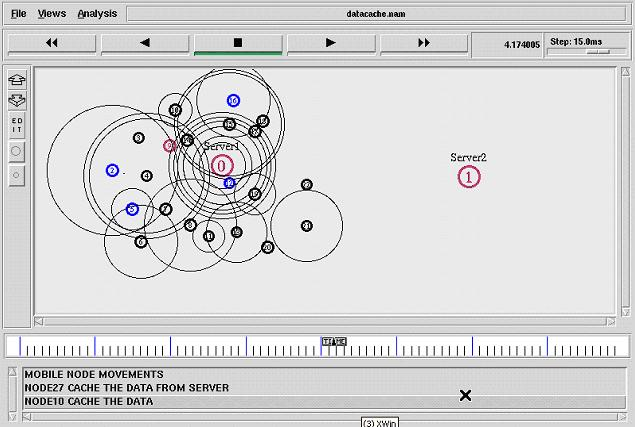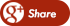﻿ How to make AODV routing protocol simulation by using NS2?Back

## How to make AODV routing protocol simulation by using NS2?

By Admin onAd hoc On-Demand Distance Vector (AODV)

The Network Simulator (NS-2) is a most widely used network simulator. It has the capabilities to simulate a range of networks including wired andwireless networks. In this tutorial, we present the implementation of Ad Hoc On-Demand Distance Vector (AODV) Protocol in NS-2.This tutorial is targeted to the novice user who wants to understand the implementation ofAODV Protocol in NS-2.The step by step process happening in AODV network simulation in NS2

1. In the TCL script, when the user configures AODV as a routing protocol by using the command,

\$ns node-config -adhocRouting AODV

the pointer moves to the “start” and this “start” moves the pointer to the Command function of AODVprotocol.

2. In the Command function, the user can find two timers in the “start”

* btimer.handle((Event*) 0);

* htimer.handle((Event*) 0);

3. Let’s consider the case of htimer, the flow points toHelloTimer::handle(Event*) function and the user can see the following lines:

agent -> sendHello();

double interval = MinHelloInterval + ((MaxHelloInterval - Min-HelloInterval) * Random::uniform());

assert(interval -> = 0);

Scheduler::instance().schedule(this, &intr, interval);

These lines are calling the sendHello() function by setting the appropriate interval of Hello Packets.

4. Now, the pointer is in AODV::sendHello() function and the user can seeScheduler::instance().schedule(target , p, 0.0) which will schedule the packets.

5. In the destination node AODV::recv(Packet*p, Handler*) is called, but actually this is done after the node is receiving a packet.

6. AODV::recv(Packet*p, Handler*) function then calls therecvAODV(p) function.

7. Hence, the flow goes to the AODV::recvAODV(Packet *p) function, which will check different packets types and call the respective function.

8. In this example, flow can go to case

AODVTYPE HELLO:

recvHello(p);

break;

9. Finally, in the recvHello() function, the packet is received.

Here you can download the following tcl file of aodv protocol

simple-wireless.tcl